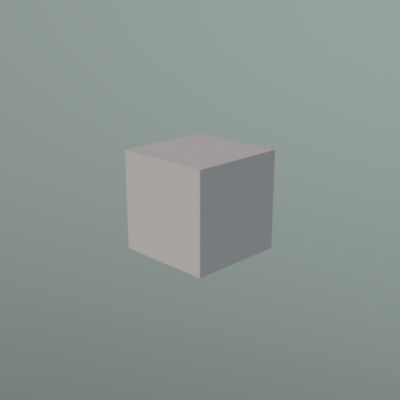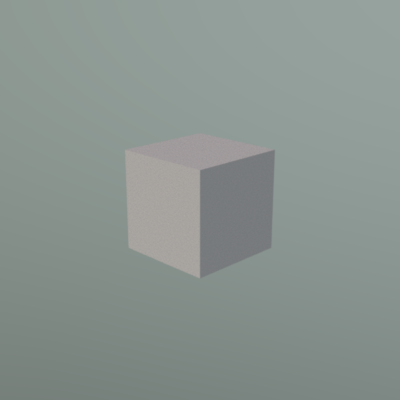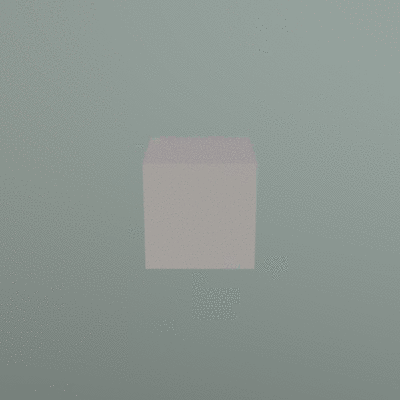# 3D Rendering with Mitsuba

In this lesson, we will be looking at how we can use Python to generate the input to the three-dimensional rendering program called Mitsuba. We will not be investigating Mitsuba itself (the software has fantastic documentation), but rather how Python can allow us to render sets of images through Mitsuba in an easy and flexible way.

Objectives

• Be able to generate an XML file for input into Mitsuba using Python.
• Use Python loops to generate animations using Mitsuba.

## Mitsuba input

Our goal will first be to replicate the Mitsuba input used to generate the cube shown below:The following is the handwritten XML source used to generate the cube image:

```<?xml version="1.0" ?>
<scene version="0.5.0">
<sensor type="perspective">
<transform name="toWorld">
<lookat origin="0, 1, -3" target="0, 0, 0" up="0, 1, 0"/>
</transform>
<sampler type="ldsampler">
<integer name="sampleCount" value="128"/>
</sampler>
<film type="ldrfilm">
<boolean name="banner" value="false"/>
<integer name="width" value="400"/>
<integer name="height" value="400"/>
</film>
</sensor>
<shape type="cube">
<transform name="toWorld">
<scale value="0.25"/>
<rotate angle="45" y="1"/>
</transform>
</shape>
</scene>
```

## Generating XML files using Python

We want to be able to generate the above XML file programmatically using Python.

The first step is to import the Python XML package:

```import xml.etree.ElementTree as etree
```

Now, we want to use this functionality to “build up” the tree structure present in the XML file. If we inspect the XML tree, we can see that the top of the tree contains `<scene version="0.5.0">`. We begin our implementation in Python by defining:

```import xml.etree.ElementTree as etree

scene = etree.Element("scene", version="0.5.0")
```

As you can see, we have created an XML ‘element’ named `"scene"`, and specified the property `version` to have the value `"0.5.0"`. We have assigned this element to a variable named `scene`.

In the XML file, we can see that the first ‘branch’ under the ‘scene’ tree is for the ‘sensor’ (`<sensor type="perspective">`). We can create this branch by using the `etree.SubElement` function:

```import xml.etree.ElementTree as etree

scene = etree.Element("scene", version="0.5.0")

sensor = etree.SubElement(
scene,
"sensor",
type="perspective"
)
```

The first argument is the element for which we want to make a sub-element; in this case, it is the root ‘scene’ element that was stored in the variable `scene`.

Next in the XML file is another sub-element (`<transform name="toWorld">`), but this time its immediate ‘parent’ is the ‘sensor’ sub-element that was stored in the variable `sensor`. We can use the same strategy to create this sub-element in Python:

```import xml.etree.ElementTree as etree

scene = etree.Element("scene", version="0.5.0")

sensor = etree.SubElement(
scene,
"sensor",
type="perspective"
)

sensor_transform = etree.SubElement(
sensor,
"transform",
name="toWorld"
)
```

Next is another sub-element (`<lookat origin="0, 1, -3" target="0, 0, 0" up="0, 1, 0"/>`), whose parent is stored in the `sensor_transform` variable. Because this sub-element doesn’t have any ‘children’, we do not need to store it in a variable:

```import xml.etree.ElementTree as etree

scene = etree.Element("scene", version="0.5.0")

sensor = etree.SubElement(
scene,
"sensor",
type="perspective"
)

sensor_transform = etree.SubElement(
sensor,
"transform",
name="toWorld"
)

etree.SubElement(
sensor_transform,
"lookat",
origin="0, 1, -3",
target="0, 0, 0",
up="0, 1, 0"
)
```

This sequence is pretty much all there is to putting together an XML tree. Now, we repeat the strategy for the rest of the required elements:

```import xml.etree.ElementTree as etree

scene = etree.Element("scene", version="0.5.0")

sensor = etree.SubElement(
scene,
"sensor",
type="perspective"
)

sensor_transform = etree.SubElement(
sensor,
"transform",
name="toWorld"
)

etree.SubElement(
sensor_transform,
"lookat",
origin="0, 1, -3",
target="0, 0, 0",
up="0, 1, 0"
)

sensor_sampler = etree.SubElement(
sensor,
"sampler",
type="ldsampler"
)

etree.SubElement(
sensor_sampler,
"integer",
name="sampleCount",
value="128"
)

sensor_film = etree.SubElement(
sensor,
"film",
type="ldrfilm"
)

etree.SubElement(
sensor_film,
"boolean",
name="banner",
value="false"
)

etree.SubElement(
sensor_film,
"integer",
name="width",
value="400"
)

etree.SubElement(
sensor_film,
"integer",
name="height",
value="400"
)

cube = etree.SubElement(
scene,
"shape",
type="cube"
)

cube_transform = etree.SubElement(
cube,
"transform",
name="toWorld"
)

etree.SubElement(
cube_transform,
"scale",
value="0.25"
)

etree.SubElement(
cube_transform,
"rotate",
angle="45",
y="1"
)
```

OK, now we have our XML tree defined and can be accessed through the root `scene` variable. Let’s have a look at its contents by using the `etree.tostring` function:

```import xml.etree.ElementTree as etree

scene = etree.Element("scene", version="0.5.0")

sensor = etree.SubElement(
scene,
"sensor",
type="perspective"
)

sensor_transform = etree.SubElement(
sensor,
"transform",
name="toWorld"
)

etree.SubElement(
sensor_transform,
"lookat",
origin="0, 1, -3",
target="0, 0, 0",
up="0, 1, 0"
)

sensor_sampler = etree.SubElement(
sensor,
"sampler",
type="ldsampler"
)

etree.SubElement(
sensor_sampler,
"integer",
name="sampleCount",
value="128"
)

sensor_film = etree.SubElement(
sensor,
"film",
type="ldrfilm"
)

etree.SubElement(
sensor_film,
"boolean",
name="banner",
value="false"
)

etree.SubElement(
sensor_film,
"integer",
name="width",
value="400"
)

etree.SubElement(
sensor_film,
"integer",
name="height",
value="400"
)

cube = etree.SubElement(
scene,
"shape",
type="cube"
)

cube_transform = etree.SubElement(
cube,
"transform",
name="toWorld"
)

etree.SubElement(
cube_transform,
"scale",
value="0.25"
)

etree.SubElement(
cube_transform,
"rotate",
angle="45",
y="1"
)

print etree.tostring(scene, "utf-8")
```
```<scene version="0.5.0"><sensor type="perspective"><transform name="toWorld"><lookat origin="0, 1, -3" target="0, 0, 0" up="0, 1, 0" /></transform><sampler type="ldsampler"><integer name="sampleCount" value="128" /></sampler><film type="ldrfilm"><boolean name="banner" value="false" /><integer name="width" value="400" /><integer name="height" value="400" /></film></sensor><shape type="cube"><transform name="toWorld"><scale value="0.25" /><rotate angle="45" y="1" /></transform></shape></scene>
```

As you can see, this is not terribly human-readable—it is just one long set of characters. Fortunately, we can use some other Python functionality to ‘prettify’ this raw XML:

```import xml.etree.ElementTree as etree
import xml.dom.minidom

scene = etree.Element("scene", version="0.5.0")

sensor = etree.SubElement(
scene,
"sensor",
type="perspective"
)

sensor_transform = etree.SubElement(
sensor,
"transform",
name="toWorld"
)

etree.SubElement(
sensor_transform,
"lookat",
origin="0, 1, -3",
target="0, 0, 0",
up="0, 1, 0"
)

sensor_sampler = etree.SubElement(
sensor,
"sampler",
type="ldsampler"
)

etree.SubElement(
sensor_sampler,
"integer",
name="sampleCount",
value="128"
)

sensor_film = etree.SubElement(
sensor,
"film",
type="ldrfilm"
)

etree.SubElement(
sensor_film,
"boolean",
name="banner",
value="false"
)

etree.SubElement(
sensor_film,
"integer",
name="width",
value="400"
)

etree.SubElement(
sensor_film,
"integer",
name="height",
value="400"
)

cube = etree.SubElement(
scene,
"shape",
type="cube"
)

cube_transform = etree.SubElement(
cube,
"transform",
name="toWorld"
)

etree.SubElement(
cube_transform,
"scale",
value="0.25"
)

etree.SubElement(
cube_transform,
"rotate",
angle="45",
y="1"
)

rough_string = etree.tostring(scene, "utf-8")
reparsed = xml.dom.minidom.parseString(rough_string)

reparsed_pretty = reparsed.toprettyxml(indent=" " * 4)

print reparsed_pretty
```

Now, we can inspect the output and see that it replicates our original handwritten XML file:

```<?xml version="1.0" ?>
<scene version="0.5.0">
<sensor type="perspective">
<transform name="toWorld">
<lookat origin="0, 1, -3" target="0, 0, 0" up="0, 1, 0"/>
</transform>
<sampler type="ldsampler">
<integer name="sampleCount" value="128"/>
</sampler>
<film type="ldrfilm">
<boolean name="banner" value="false"/>
<integer name="width" value="400"/>
<integer name="height" value="400"/>
</film>
</sensor>
<shape type="cube">
<transform name="toWorld">
<scale value="0.25"/>
<rotate angle="45" y="1"/>
</transform>
</shape>
</scene>
```

Our final step is to save the contents of `reparsed_pretty` into an XML file that we can then use in Mitsuba. To do so, we use the `with` construct to create and open a file called `cube_python.xml` for writing (`"w"`), and write the contents of `reparsed_pretty` into the file:

```import xml.etree.ElementTree as etree
import xml.dom.minidom

scene = etree.Element("scene", version="0.5.0")

sensor = etree.SubElement(
scene,
"sensor",
type="perspective"
)

sensor_transform = etree.SubElement(
sensor,
"transform",
name="toWorld"
)

etree.SubElement(
sensor_transform,
"lookat",
origin="0, 1, -3",
target="0, 0, 0",
up="0, 1, 0"
)

sensor_sampler = etree.SubElement(
sensor,
"sampler",
type="ldsampler"
)

etree.SubElement(
sensor_sampler,
"integer",
name="sampleCount",
value="128"
)

sensor_film = etree.SubElement(
sensor,
"film",
type="ldrfilm"
)

etree.SubElement(
sensor_film,
"boolean",
name="banner",
value="false"
)

etree.SubElement(
sensor_film,
"integer",
name="width",
value="400"
)

etree.SubElement(
sensor_film,
"integer",
name="height",
value="400"
)

cube = etree.SubElement(
scene,
"shape",
type="cube"
)

cube_transform = etree.SubElement(
cube,
"transform",
name="toWorld"
)

etree.SubElement(
cube_transform,
"scale",
value="0.25"
)

etree.SubElement(
cube_transform,
"rotate",
angle="45",
y="1"
)

rough_string = etree.tostring(scene, "utf-8")
reparsed = xml.dom.minidom.parseString(rough_string)

reparsed_pretty = reparsed.toprettyxml(indent=" " * 4)

with open("cube_python.xml", "w") as cube_xml:
cube_xml.write(reparsed_pretty)
```

## Executing Mitsuba through Python

The above section has produced an XML file called `cube_python.xml` that can then be passed manually into mitsuba to render the image:

```mitsuba cube_python.xml
```

However, it would be handy if we could do this directly from Python rather than having to type the command. We can do so using the `subprocess` functionality in Python. The first step is, as usual, to import it:

```import subprocess
```

Now, we need to specify the command that we wish to run. To do so, we need to form a list of strings where each element corresponds to the components of the command that we wish to run:

```import subprocess

cmd = ["mitsuba", "cube_python.xml"]
```

Tip

Specifying the command as `mitsuba` will depend on your operating system ‘knowing’ where that executable is. If not using linux, you will probably need to specify it more directly; something like `"c:\\Mitsuba 0.5.0\\mitsuba.exe"` for Windows and `"/Appplications/Mitsuba.app/Contents/MacOS/mitsuba"` for Mac.

To execute the command, we can use the `subprocess.check_output` function:

```import subprocess

cmd = ["mitsuba", "cube_python.xml"]

cmd_out = subprocess.check_output(cmd)
```

The output from this command is stored as a string in the variable `cmd_out`, which we can inspect:

```import subprocess

cmd = ["mitsuba", "cube_python.xml"]

cmd_out = subprocess.check_output(cmd)

print cmd_out
```
```2017-02-20 13:25:25 INFO  main [mitsuba.cpp:275] Mitsuba version 0.5.0 (Linux, 64 bit), Copyright (c) 2014 Wenzel Jakob
2017-02-20 13:25:25 INFO  main [mitsuba.cpp:377] Parsing scene description from "cube_python.xml" ..
2017-02-20 13:25:25 INFO  ren0 [KDTreeBase] Constructing a SAH kd-tree (12 primitives) ..
2017-02-20 13:25:25 INFO  ren0 [KDTreeBase] Finished -- took 0 ms.
2017-02-20 13:25:25 WARN  ren0 [Scene] No emitters found -- adding sun & sky.
2017-02-20 13:25:25 INFO  ren0 [EnvironmentMap] Precomputing data structures for environment map sampling (515.0 KiB)
2017-02-20 13:25:25 INFO  ren0 [EnvironmentMap] Done (took 0 ms)
2017-02-20 13:25:25 INFO  ren0 [SamplingIntegrator] Starting render job (400x400, 128 samples, 8 cores, SSE2 enabled) ..

Rendering: [++++++++                                    ] (1.0s, ETA: 4.2s)  
Rendering: [+++++++++++++++++++++++++                   ] (2.0s, ETA: 1.4s)  
Rendering: [++++++++++++++++++++++++++++++++++++++++++++] (2.8s, ETA: 0.0s)  
2017-02-20 13:25:28 INFO  ren0 [RenderJob] Render time: 2.9150s
2017-02-20 13:25:28 INFO  ren0 [LDRFilm] Writing image to "/home/damien/venv_study/psych_programming/source/vision/mitsuba/cube_python.png" ..
2017-02-20 13:25:28 INFO  main [statistics.cpp:142] Statistics:
------------------------------------------------------------
-  plugins/cube.so [Cube intersection primitive]
-  plugins/diffuse.so [Smooth diffuse BRDF]
-  plugins/direct.so [Direct illumination integrator]
-  plugins/envmap.so [Environment map]
-  plugins/gaussian.so [Gaussian reconstruction filter]
-  plugins/lanczos.so [Lanczos Sinc filter]
-  plugins/ldrfilm.so [Low dynamic range film]
-  plugins/ldsampler.so [Low discrepancy sampler]
-  plugins/perspective.so [Perspective camera]
-  plugins/sky.so [Skylight emitter]
-  plugins/sphere.so [Sphere intersection primitive]
-  plugins/sunsky.so [Sun & sky emitter]

* General :
-  Normal rays traced : 22.755 M
-  Shadow rays traced : 2.275 M

* Texture system :
-  Cumulative MIP map memory allocations : 1 MiB
-  Filtered texture lookups : 72.74 % (18.21 M of 25.03 M)
-  Lookups with clamped anisotropy : 0.00 % (0.00 of 18.21 M)
------------------------------------------------------------

```

And we can also have a look at the `cube_python.png` file that is produced:## Using Python to create an animation via Mitsuba

Finally, we will look at why it is useful to do this via Python rather than via the handwritten XML file—we seem to have just made the process more complicated!

The power of doing it through Python, for simple scenes like this, really becomes evident when we want to animate aspects of the scene. For this example, we will want to produce an animation of a rotating cube. More specifically, we will want the cube to complete a full revolution in a few seconds. To create a video that runs at 15 frames per second, we will need to generate 49 frames—in each, the cube will have a slightly different rotation. We don’t want to have to make all these XML files by hand...

Instead, we can make a couple of minor adaptations to our Python code to generate all of our frames. First, we specify the rotations via a chunk of code like:

```import numpy as np

rotations = np.linspace(
start=0.0,
stop=360.0,
num=50 - 1,
endpoint=False
)
```

Now we have the required rotation for each of our frames, we can alter our XML creation and mitsuba rendering code such that it inserts the appropriate rotation value into each frame:

```import xml.etree.ElementTree as etree
import xml.dom.minidom

import subprocess

import numpy as np

rotations = np.linspace(
start=0.0,
stop=360.0,
num=50 - 1,
endpoint=False
)

for (frame, rotation) in enumerate(rotations, 1):

scene = etree.Element("scene", version="0.5.0")

sensor = etree.SubElement(
scene,
"sensor",
type="perspective"
)

sensor_transform = etree.SubElement(
sensor,
"transform",
name="toWorld"
)

etree.SubElement(
sensor_transform,
"lookat",
origin="0, 1, -3",
target="0, 0, 0",
up="0, 1, 0"
)

sensor_sampler = etree.SubElement(
sensor,
"sampler",
type="ldsampler"
)

etree.SubElement(
sensor_sampler,
"integer",
name="sampleCount",
value="128"
)

sensor_film = etree.SubElement(
sensor,
"film",
type="ldrfilm"
)

etree.SubElement(
sensor_film,
"boolean",
name="banner",
value="false"
)

etree.SubElement(
sensor_film,
"integer",
name="width",
value="400"
)

etree.SubElement(
sensor_film,
"integer",
name="height",
value="400"
)

cube = etree.SubElement(
scene,
"shape",
type="cube"
)

cube_transform = etree.SubElement(
cube,
"transform",
name="toWorld"
)

etree.SubElement(
cube_transform,
"scale",
value="0.25"
)

etree.SubElement(
cube_transform,
"rotate",
angle="{angle:.12f}".format(angle=rotation),
y="1"
)

rough_string = etree.tostring(scene, "utf-8")
reparsed = xml.dom.minidom.parseString(rough_string)

reparsed_pretty = reparsed.toprettyxml(indent=" " * 4)

with open("cube_python.xml", "w") as cube_xml:
cube_xml.write(reparsed_pretty)

cmd = [
"mitsuba",
"-o", "cube_python_{n:02d}.png".format(n=frame),
"cube_python.xml"
]

cmd_out = subprocess.check_output(cmd)
```

There are a few features to note in the above code:

• Notice how all the XML file creation and mitsuba execution code is now nested underneath a `for` loop.
• The loop uses the `enumerate` function to set both the rotation value (`rotation`) and the frame number (`frame`) for each iteration of the loop.
• Where we specify the cube rotation in the XML generation, we use string formatting to convert the contents of the variable `rotation` (a number) to a string representing a decimal, to 12 places (`"{angle:.12f}".format(angle=rotation)`).
• Because we don’t care about having a separate XML file for each rotation, we simply overwrite `cube_python.xml` on every iteration through the loop. However, since we want to generate a different image on every iteration, we use the `-o` flag in the mitsuba command to include the frame number in the image filename.
• We use a similar strategy to how we specified the rotation to specify the frame number in the image file. However, here we want to convert an integer to a representation with two characters, including a leading zero if required (`"cube_python_{n:02d}.png".format(n=frame)`).

Now we have rendered each of our frames, we can use the sort of knowledge we have from the Drawing—images lesson to produce an animation:

```import psychopy.visual
import psychopy.event

win = psychopy.visual.Window(
size=[400, 400],
units="pix",
fullscr=False
)

frames = []

# store each frame as a separate 'ImageStim'
for frame_num in xrange(1, 50):

frame = psychopy.visual.ImageStim(
win=win,
units="pix",
size=[400, 400],
image="cube_python_{n:02d}.png".format(n=frame_num)
)

frames.append(frame)

i_frame_to_draw = 0

keep_going = True

while keep_going:

# because we rendered as 15fps, draw each frame x4 (assuming 60Hz
# refresh)
for _ in xrange(4):
frames[i_frame_to_draw].draw()
win.flip()

keys = psychopy.event.getKeys()

keep_going = (len(keys) == 0)

# increment the frame to draw, wrapping around when necessary
i_frame_to_draw = (i_frame_to_draw + 1) % len(frames)

win.close()
```